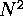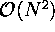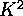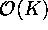Next: Combinations of the methods. Up: Relations and differences between Previous: Relations of the mappings

#### Computational properties.

It is common to all MDS methods that they do not construct an explicit mapping function, but instead the projections of all samples are computed in a simultaneous optimization process. Thus, new samples cannot be projected without recomputing the whole projection, at least not accurately.

At least in the case of Sammon's mapping this problem may be alleviated somewhat by computing the projection with a multilayer perceptron-type network which learns to minimize the cost function. This can be done by using a learning rule that is analogous to the error backpropagation algorithm [Mao and Jain, 1995]. The method does not necessarily reduce the computational complexity of the original algorithm since the backpropagation learning rule is computationally very intensive, but it generalizes the mapping to new samples. An alternative approach is to use a radial basis function (RBF) network to construct the distance-preserving mapping [Webb, 1995]; this could be a promising alternative to the original MDS methods.

The optimization of the cost functions of the MDS methods requires comparisons between all pairs of vectors, whereby their computational complexity is of the order of,, where N is the number of data items. The computational complexity of the SOM is, where K is the number of map units: each learning step requirescomputations, and to achieve a sufficient statistical accuracy the number of iterations should be at least some multiple of K.

If the size of the map is of the order of the number of input samples as in Publication 2, the computational complexities of the methods are of the same order of magnitude. If reduction of complexity is needed, the resolution of the SOM can be reduced by using a smaller map. It is also possible to use faster versions of the algorithm, discussed in Section 6.4.4, or a parallel implementation. In Publications 3, 4, and 5 a massively parallel CNAPS neurocomputer was used to perform part of the SOM computations.

It has been proposed [Chang and Lee, 1973] that the computational complexity of Sammon's mapping could be reduced by applying it only to a representative subset of the total data set. The rest of the data items could then be mapped one by one, thereby only trying to optimize their distances from the fixed subset. This method is not as accurate as the original, however.

Besides the computational complexity, the existence of local minima in the cost functions may also cause problems. On the basis of empirical experience Sammon's mapping can easily get stuck at local optima (cf. Mao and Jain, 1995, and Ripley, 1996), and in practice the computation of the projection must be repeated several times starting from different initial configurations. The SOM algorithm, on the other hand, seems to be quite robust if the learning is begun with a wide neighborhood, which then shrinks gradually to its final narrow form [Kohonen, 1995c]. There may be some differences in the resulting maps, depending on their initial state and the stochastic input sequence, however, and the effect of the differences in the actual use of the maps should be quantified empirically. The measures proposed in Publication 7 might thereby be of help.Next: Combinations of the methods. Up: Relations and differences between Previous: Relations of the mappings

Sami Kaski
Mon Mar 31 23:43:35 EET DST 1997